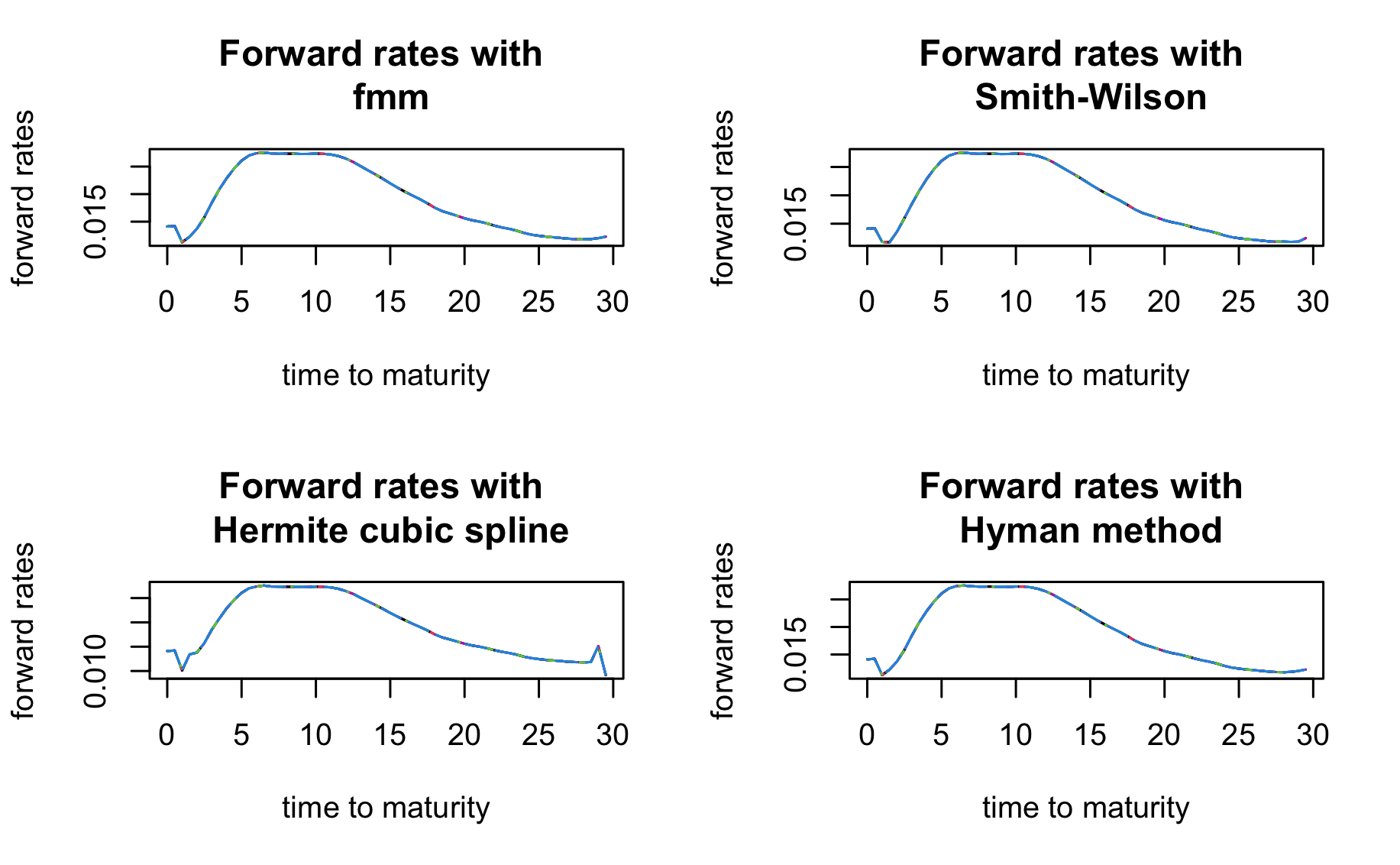Contents

• 1 - news
• 2 - examples

# 1 - news

Version 0.6.2 of ESGtoolkit is now available:

It is now possible to use Hermite cubic spline and Smith-Wilson methods for yield curve interpolation.

Starting with version 1.0.0, the next version, ESGtoolkit will be renamed as esgtoolkit or something similar, as I’m removing all my packages from CRAN.

A Python version of esgtoolkit will also be available soon on Techtonique.

# 2 - examples

Single curve

library(ESGtoolkit)

# Yield to maturities
txZC <- c(0.01422,0.01309,0.01380,0.01549,0.01747,0.01940,0.02104,0.02236,0.02348,
0.02446,0.02535,0.02614,0.02679,0.02727,0.02760,0.02779,0.02787,0.02786,0.02776
,0.02762,0.02745,0.02727,0.02707,0.02686,0.02663,0.02640,0.02618,0.02597,0.02578,0.02563)

# Observed time to maturities
u <- 1:30

par(mfrow=c(2,2))
fwd1 <- ESGtoolkit::esgfwdrates(in.maturities = u, in.zerorates = txZC,
n = 10, horizon = 20,
out.frequency = "semi-annual", method = "fmm")
matplot(as.vector(time(fwd1)), fwd1, type = 'l',
main = "Forward rates with \n fmm",
xlab = "time to maturity", ylab = "forward rates")

fwd2 <- ESGtoolkit::esgfwdrates(in.maturities = u, in.zerorates = txZC,
n = 10, horizon = 20,
out.frequency = "semi-annual", method = "SW")
matplot(as.vector(time(fwd2)), fwd2, type = 'l',
main = "Forward rates with \n Smith-Wilson",
xlab = "time to maturity", ylab = "forward rates")

fwd3 <- ESGtoolkit::esgfwdrates(in.maturities = u, in.zerorates = txZC,
n = 10, horizon = 20,
out.frequency = "semi-annual", method = "HCSPL")
matplot(as.vector(time(fwd2)), fwd3, type = 'l',
main = "Forward rates with \n Hermite cubic spline",
xlab = "time to maturity", ylab = "forward rates")

fwd4 <- ESGtoolkit::esgfwdrates(in.maturities = u, in.zerorates = txZC,
n = 10, horizon = 20,
out.frequency = "semi-annual", method = "hyman")
matplot(as.vector(time(fwd4)), fwd4, type = 'l',
main = "Forward rates with \n Hyman method",
xlab = "time to maturity", ylab = "forward rates")